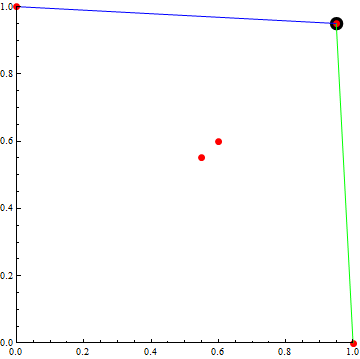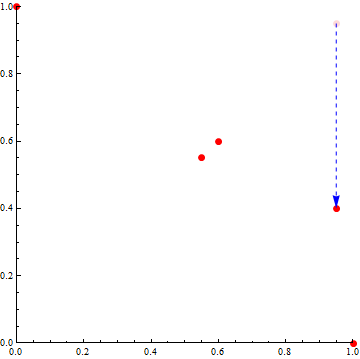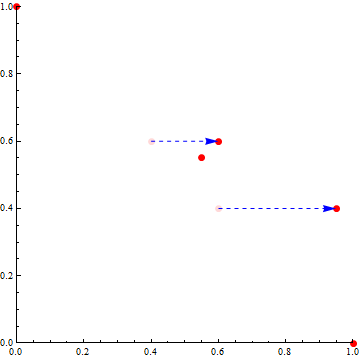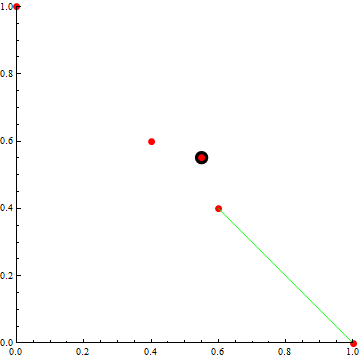# 15

Personal Blog

This is a simple picture proof to show that if there is any decision process that will find a Pareto outcome for two people, it must be that liars will prosper: there are some circumstances where you would come out ahead if you were to lie about your utility function.

Apart from Pareto, the only other assumption it needs are that if the data is perfectly symmetric, then the outcome will be symmetric as well. We won't even need to use affine independence or other scalings of utility functions.

Now, given Pareto-optimality, symmetry allows us to solve symmetric problems by taking the unique symmetric Pareto option. Two such symmetric problems presented here, and in one of them, one of the two players must be able to prosper by lying.

So first assume Pareto-optimality, symmetry, and (by contradiction) that liars don't prosper. The players are x and y, and we will plot their utilities in the (x,y) plane. The first setup is presented in this figure:There are five pure choices here, of utilities (0,1), (0.95,0.95), (1,0) and the non-Pareto optimal ones at (0.6,0.6) and (0.55,0.55). By symmetry and Pareto-optimality, we know that the outcome has to be (0.95,0.95).

Now player y is going to lie. If Player y can force the outcome off the green line and onto the blue line, then he will profit by lying. He is going to claim that the choice (0.95,0.95) actually only gives him a utility of (0.4), and so is at (0.95, 0.4). This results in this diagram:The Pareto optimal boundary of this is:Now, the new outcome must be on the green segment somewhere (including the end points). Or else, as we have seen, player y will have profited by lying. Got that? If liars don't prosper, then the outcome for the above diagram must be on the green segment.

Now let's consider a new setup, namely:This has choices with utility (1,0), (0.55,0.55), (0,1) and non-Pareto optimal choices (0.4,0.6) and (0.6,0.4). It is symmetric, so the outcome must be (0.55, 0.55) by Pareto-optimality.

Now it's player x's chance to lie. She will lie on two of her choices, claiming that (0.4,0.6) is actually at (0.6,0.6) and that (0.6,0.4) is actually at (0.95, 0.4):You will no doubt be astounded and amazed to realise that this setup is precisely the same as in the third figure! Now, we know that the outcome for that must lie along the green line between (1,0) and (0.95,0.4). Translating that green line back into the real utility for x, you get:Any point on that line is better, from x's perspective, than the standard outcome (0.55,0.55) (she will get at least 0.6 in utility on the green line). So, if we accept Pareto-optimality and symmetry, then one of the players has to be able to profit by lying in certain situations.

Pareto-optimality is required: if you waive that condition, then some non-Pareto solutions such as "flip a coin, and the winner gets to decide the outcome" do not allow liars to prosper.

Personal Blog